# How to add and subtract dates, days, weeks, months and years in Excel

In this tutorial, you will find a variety of useful formulas to add and subtract dates in Excel, such as subtracting two dates, adding days, weeks, months and years to a date, and more.

If you have been following our tutorials to working with dates in Excel, you already know an array of formulas to calculate different time units such as weekdays, weeks, months and years.

When analyzing the date information in your worksheets, you are likely to perform some arithmetic operations with those dates too. This tutorial explains a few formulas for adding and subtracting dates in Excel that you may find useful.

## How to subtract dates in Excel

Supposing you have two dates in cells A2 and B2, and now you want to subtract one date from the other to know how many days are between these dates. As is often the case in Excel, the same result can be achieved in several ways.

#### Example 1. Subtract one date from the other directly

As you probably know, Microsoft Excel stores each date as a unique serial numbers beginning with 1 that represents January 1, 1900. So, you are actually subtracting two numbers, and an ordinary arithmetic operation works without a hitch:

`=B2-A2`

#### Example 2. Subtract dates using Excel DATEDIF function

If the above formula looks too plain, you can achieve the same result in a guru-like way by using Excel's DATEDIF function:

`=DATEDIF(A2, B2, "d")`

The following screenshot demonstrates that both calculations return identical results, except for row 4 where the DATEDIF function returns the #NUM! error. Let's figure out why that happens.

When you subtract a more recent date (6-May-2015) from an earlier date (1-May-2015), the subtraction operation returns a negative number (-5) exactly as it should. The syntax of the Excel DATEDIF function, however, does not allow the start date to be greater than the end date and therefore it returns an error.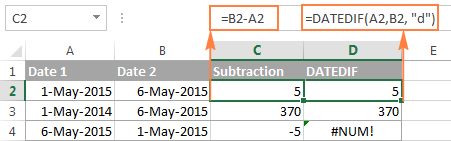#### Example 3. Subtract a date from the current date

To subtract a date from today's date, you can employ either of the above formulas. Just use the TODAY() function instead of date 1:

`=TODAY()-A2`

or

`=DATEDIF(A2,TODAY(), "d")`

Like in the previous example, both formulas work fine when today's date is greater than the date you are subtracting from it, otherwise DATEDIF fails: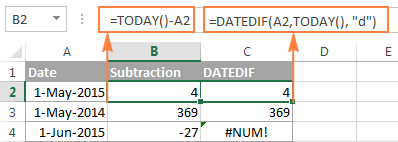#### Example 4. Subtracting dates with Excel DATE function

If you prefer to supply the dates directly in the formula, then enter each date using the DATE(year, month, day) function and then subtract one date from the other.

For instance, the following formula subtracts 15-May-2015 from 20-May-2015 and returns the difference of 5 days:

`=DATE(2015, 5, 20) - DATE(2015, 5, 15)`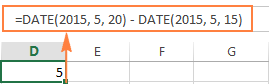Wrapping up, when it comes to subtracting dates in Excel and you want to find out how many days are between two dates, it makes sense to go with the easiest and most obvious option - simply subtract one date directly from another.

If you are looking to count the number of months or years between two dates, then the DATEDIF function is the only possible solution and you will find a few formula examples in the next article that will cover this function in full details.

Now that you know how to subtract two dates, let's see how you can add or subtract days, months, or years to a given date. There are a number of Excel functions suitable for this purpose, and which one you use depends on which unit you want to add or subtract.

## How to subtract or add days to date in Excel

If you have a date in some cell or a list of dates in a column, you can add or subtract a certain number of days to those dates using a corresponding arithmetic operation.

#### Example 1. Adding days to a date in Excel

The general formula to add a specified number of days to a date in as follows:

Date + N days

The date can be entered in several ways:

• As a cell reference, e.g. `=A2 + 10`
• Using the DATE(year, month, day) function, e.g. `=DATE(2015, 5, 6) + 10`
• As a result of another function. For example, to add a given number of days to the current date, use the TODAY() function: `=TODAY()+10`

The following screenshot demonstrates the above formulas in action. The current date at the moment of writing was 6 May, 2015: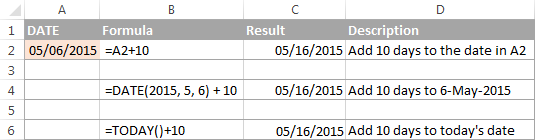Note. The result of the above formulas is a serial number representing the date. To get it displayed as a date, select the cell(s) and press Ctrl+1 to open the Format Cells dialog. On the Number tab, select Date in the Category list, and then choose the date format you want. For the detailed steps, please see How to change date format in Excel.

#### Example 2. Subtracting days from a date in Excel

To subtract a given number of days from a certain date, you perform a usual arithmetic operation again. The only difference from the previous example is that you type the minus sign instead of plus :)

Date - N days

Here are a few formula examples:

• `=A2-10`
• `=DATE(2015, 5, 6)-10`
• `=TODAY()-10`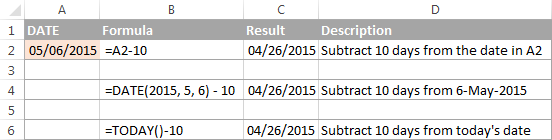## How to add or subtract weeks to date

In case you want to add or subtract whole weeks to a certain date, you can use the same formulas as for adding / subtracting days, and simply multiply the number of weeks by 7:

Adding weeks to a date in Excel:

cell+ N weeks * 7

For example, you add 3 weeks to the date in A2, use the following formula: `=A2+3*7`.

Subtracting weeks from date in Excel:

cell - N weeks * 7

To subtract 2 weeks from today's date, you write `=TODAY()-2*7`.

## How to add / subtract months to date in Excel

If you want to add or subtract a certain number of whole months to a date, you can employ either the DATE or EDATE function, as demonstrated below.

#### Example 1. Add months to a date with Excel DATE function

Taking a list of dates in column A for example, type the number of dates you want to add (positive number) or subtract (negative number) in some cell, say C2.

Enter the following formula in cell B2 and then drag the corner of the cell all the way down to copy the formula to other cells:

`=DATE(YEAR(A2), MONTH(A2) + \$C\$2, DAY(A2))`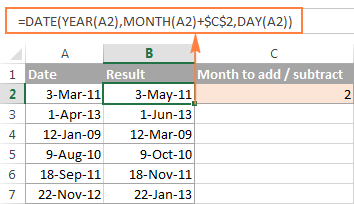Now, let's see what the function is actually doing. The logic behind the formula is obvious and straightforward. The DATE(year, month, day) function takes the following arguments:

• the year of the date in cell A2;
• the month of the date in A2 + the number of months you specified in cell C2, and
• the day of the date in A2.

Yep, it's that simple :) If you type a negative number in C2, the formula will subtract months instead of adding them: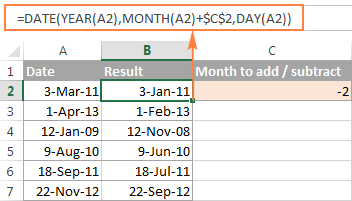Naturally, nothing prevents you from typing the minus sign directly in the formula to subtract months from a date:

`=DATE(YEAR(A2), MONTH(A2) - \$C\$2, DAY(A2))`

And of course, you can type the number of month to add or subtract in the formula instead of referring to a cell:

`=DATE(YEAR(date), MONTH(date) + N months, DAY(date))`

The real formulas could look similar to these:

• Add months to date: `=DATE(YEAR(A2), MONTH(A2) + 2, DAY(A2))`
• Subtract months from date: `=DATE(YEAR(A2), MONTH(A2) - 2, DAY(A2))`

#### Example 2. Add or subtract months to a date with Excel EDATE

Microsoft Excel provides a special function that returns a date that is a specified number of months before or after the start date - the EDATE function. It is available in all versions of Excel 2007 and higher.

In your `EDATE(start_date, months)` formulas, you supply the following 2 arguments:

• Start_date - the start date from which to count the number of months.
• Months - the number of months to add (a positive value) or subtract (a negative value).

The following formula used on our column of dates yields exactly the same results as the DATE function in the previous example: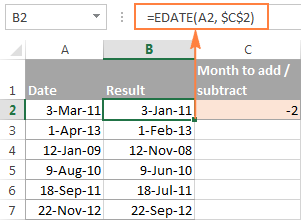When using the EDATE function, you can also specify the start date and the number of month to add / subtract directly in the formula. Dates should be entered by using the DATE function or as results of other formulas. For example:

• To add months in Excel:

`=EDATE(DATE(2015,5,7), 10)`

The formula adds 10 months to 7-May-2015.

• To subtract months in Excel:

`=EDATE(TODAY(), -10)`

The formula subtracts 10 months from today's date.

Note. The Excel EDATE function returns a serial number representing the date. To force Excel to display it as a date, you should apply the Date format to the cells with your EDATE formulas. Please see Changing the date format in Excel for the detailed steps.

## How to subtract or add years to date in Excel

Adding years to a date in Excel is done similarly to adding months. You use the DATE(year, month, day) function again, but this time you specify how many years you want to add:

DATE(YEAR(date) + N years, MONTH(date), DAY(date))

In your Excel worksheet, the formulas may look as follows:

• To add years to a date in Excel:

`=DATE(YEAR(A2) + 5, MONTH(A2), DAY(A2))`

The formula adds 5 years to the date in cell A2.

• To subtract years from a date in Excel:

`=DATE(YEAR(A2) - 5, MONTH(A2), DAY(A2))`

The formula subtracts 5 years from the date in cell A2.

If you type the number of year to add (positive number) or subtract (negative number) in some cell and then refer to that cell in the DATE function, you will get a universal formula: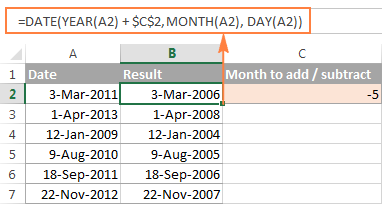## Add / subtract days, months and years to date

If you carefully observed the two previous examples, I think you have already guessed how to add or subtract a combination of years, months and days to a date in a single formula. Yep, using the good old DATE function :)

DATE(YEAR(date) + X years, MONTH(date) + Y months, DAY(date) + Z days)

To subtract years, months, days:

DATE(YEAR(date) - X years, MONTH(date) - Y months, DAY(date) - Z days)

For example, the following formula adds 2 years, 3 months and subtracts 15 days from a date in cell A2:

`=DATE(YEAR(A2) + 2, MONTH(A2) + 3, DAY(A2) - 15)`

Applied to our column of dates, the formula takes the following shape:

`=DATE(YEAR(A2) + \$C\$2, MONTH(A2) + \$D\$2, DAY(A2) + \$E\$2)`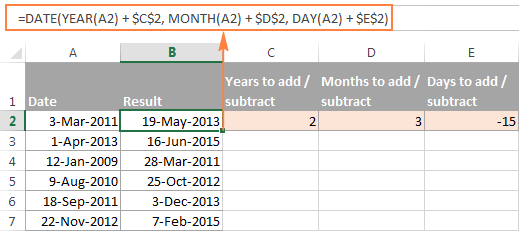## How to add and subtract times in Excel

In Microsoft Excel, you can add or subtract times using the TIME function. It lets you operate on time unites (hours, minutes and seconds) exactly in the same way as you handle years, months and days with the DATE function.

cell + TIME(hours, minutes, seconds)

To subtract time in Excel:

cell - TIME(hours, minutes, seconds)

Where A2 contains the time value you want to change.

For example, to add 2 hours, 30 minutes and 15 seconds to the time in cell A2, you can use the following formula:

`=A2 + TIME(2, 30, 15)`

If you want to add and subtract time unites within one formula, just add the minus sign to the corresponding values:

`=A2 + TIME(2, 30, -15)`

The above formula adds 2 hours and 30 minutes to the time in cell A2 and subtracts 15 seconds.

Alternatively, you can enter the time unites you want to changes in some cells, and refer to those cells in your formula:

`=A2 + TIME(\$C\$2, \$D\$2, \$E\$2)`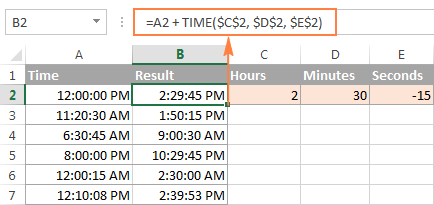If the original cells contain both date and time, the above formula works perfectly too: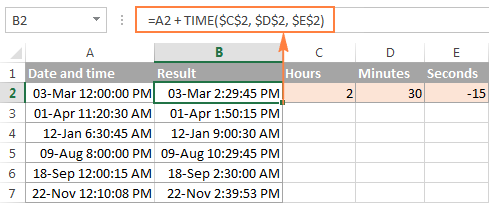## Date & Time Formula Wizard - quick way to add and subtract dates in Excel

Now that you know a bunch of different formulas to calculate dates in Excel, wouldn't you want to have just one that can do all this? Of course, such a formula can never exist. However, there exists the Date & Time Wizard that can build any formula for you on the fly, provided that you have our Ultimate Suite installed in your Excel. Here's how:

1. Select the cell in which you want to insert the formula.
2. Head to the Ablebits Tools tab, and click the Date & Time Wizard button:3. The Date & Time Wizard dialog window shows up. Depending on whether you want to add or subtract dates, switch to the corresponding tab, supply data for the formula arguments, and click the Insert Formula button.

As an example, let's add a few months to the date in cell A2. For this, you go to the Add tab, type A2 in the Enter a date box (or click in the box and select the cell on the sheet), and type the number of months to add in the Month box.

The wizard makes a formula and shows its preview in the cell. It also shows the calculated date under Formula result: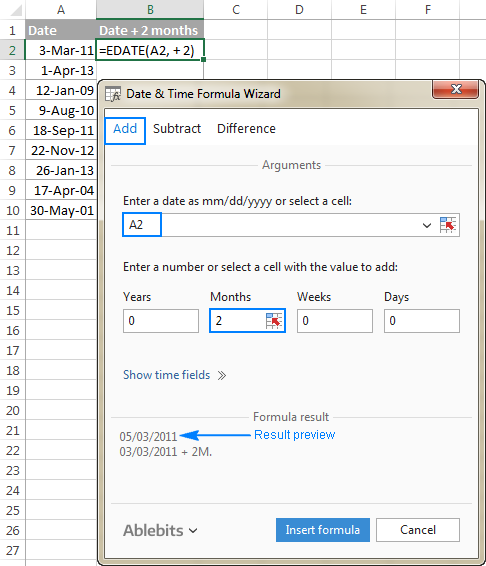If you are satisfied with the result, click the Insert formula button. Once the formula is added, you can copy it to as many cells as necessary: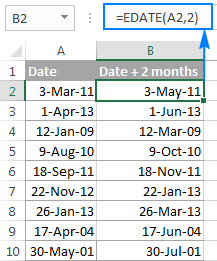That was quite a simple formula, wasn't it? Let's give the wizard something more challenging to work on. For example, let us subtract some years, months, weeks and days from the date in A2. To have it done, switch to the Subtract tab and type the numbers in the corresponding boxes. Or you can enter the units in separate cells and supply references to those cells, as shown in the screenshot below: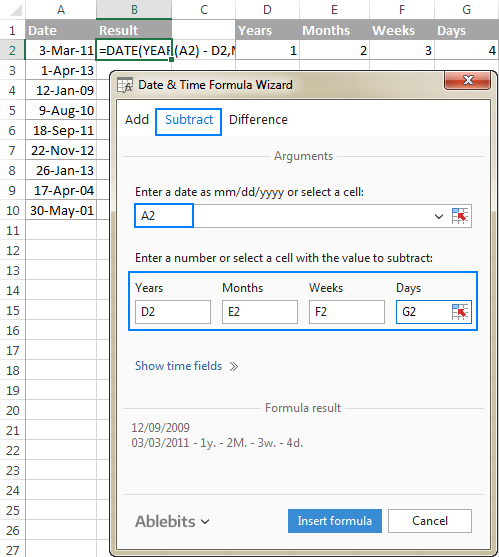Clicking the Insert formula button inputs the following formula in A2:

`=DATE(YEAR(A2)-D2,MONTH(A2)-E2,DAY(A2)-G2-F2*7)`

If you plan to copy the formula to other cells, you have to change all cell references except A2 to absolute references so that the formula copies correctly (by default, the wizard always uses relative references). To fix the reference, you simply type the \$ sign before the row and column coordinates, like this:

`=DATE(YEAR(A2)-\$D\$2,MONTH(A2)-\$E\$2,DAY(A2)-\$G\$2-\$F\$2*7)`

And get the following results: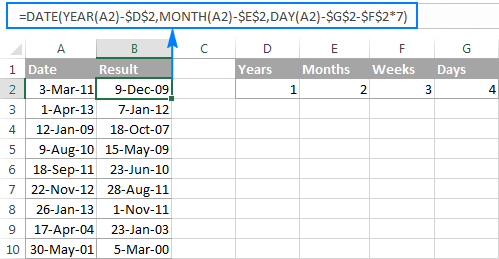Additionally, you can click the Show time fields link and add or subtract date and time units with one formula.

If you wish to play with the Date & Time Formula Wizard in your own worksheets, you are welcome to download the 14-day trial version of the Ultimate Suite.

This is how you add and subtract dates in Excel. I am hopeful you have learned a couple of useful functions today. I thank you for reading and hope to see you on our blog next week.

## You may also be interested in

1. Hello,

I am trying to create a 60 day calculator and I want to add the option to not count specific dates. What's the best way to do this? For example, I want to calculate 60 days from May 5, 2023 but I do not want it to count days listed in columns D in the 60 days.

Thank you!

• Hi! Try to use WORKDAY.INTL function. For examplle,

WORKDAY.INTL(start_date, 60, "0000000", D1:D20)

2. Hi Maam,

i am try to addition 9 hour each cell reference

example - =time(9,0,0)*5

wrong output geeting

thank you
Achyutam

• Hi!
Pay attention to the following paragraph of the article above: How to add and subtract times in Excel.
Here is an example of a formula:
=A1+time(9,0,0)

3. I need to calculate the expiration date to 12/31/****, 20 years from date of C.O date. Example given
Sale Date C.O. Date Expiration Date
6/25/2021 6/25/2021
6/28/2021 6/24/2021
6/28/2021 6/25/2021

4. PLEASE DISREGARD PREVIOUS POST! It somehow deleted part of my comment when I posted

Hi there,

Sorry if this is on your page but I am not an expert on Excel and I have read through the article a few times.

I am conditionally formatting a column on dates for expiry of First Aid Certificate. At the moment I have the following formulas:

=AND(\$E2-TODAY()>=0,\$E2-TODAY()=31) (Filled in RED)

=AND(\$E1-TODAY()>=31,\$E1-TODAY()=92,\$E1-TODAY()=184,\$E1-TODAY()<=365) (Filled in GREEN)

However I have two issues with this:
1. If I don't put =TODAY() in one of the cells in the column it fails to highlight nearby dates (eg tomorrow or the next day, 13/01/2023 and 14/01/2023)
2. If I have dates in 2024 it colour fills them based on their day and month. Eg. if I have a date 01/02/2024 it fills in the cell in ORANGE.

From what I have read it seems that you can alter the formula to include the YEAR into consideration but I am just not quite sure how to format it for future date ranges as I have above.

Thanks so much for your help.

5. Hi there,

Sorry if this is on your page but I am not an expert on Excel and I have read through the article a few times.

I am conditionally formatting a column on dates for expiry of First Aid Certificate. At the moment I have the following formulas:

=AND(\$E2-TODAY()>=0,\$E2-TODAY()=31,\$E1-TODAY()=92,\$E1-TODAY()=184,\$E1-TODAY()<=365) (Filled in GREEN)

However I have two issues with this:
1. If I don't put =TODAY() in one of the cells in the column it fails to highlight nearby dates (eg tomorrow or the next day, 13/01/2023 and 14/01/2023)
2. If I have dates in 2024 it colour fills them based on their day and month. Eg. if I have a date 01/02/2024 it fills in the cell in ORANGE.

From what I have read it seems that you can alter the formula to include the YEAR into consideration but I am just not quite sure how to format it for future date ranges as I have above.

Thanks so much for your help.

• Hi!
Sorry, it's not quite clear what you are trying to achieve. Conditions \$E1-TODAY()=92 and \$E1-TODAY()=184 cannot be met at the same time.
To understand what you want to do, give an example of the source data and the desired result.

• Sorry, Sorry, Sorry -
I'm trying hard to explain what I'm looking for - So I wrote it a little better this time

I'm trying to find or get a formula or VBA for inventory, Sheet1 (Inventory) and sheet2 (weekly Inventory)
I have the date setup on sheet1 to change by Date
Here come the problem
I will like to have the inventory to pass from Sheet1 , to Sheet2 by date.

Example:
On Friday, I sold 100 marbles and 50 table cloth, - It is on B5 and B6 on Sheet1
On Saturday I sold, 10 marbles and 10 table cloth,
I will like that information to be on Column "Friday" witch it is on sheet2 on E5 and E6,
Column Saturday is on H5 and H6, so I can Sum the entire week
keep in mind that I will use Sheet1 Inventory on the daily bases, so I will change the amount Sold at the end of the day
but don't want the info on Shee2, Column E (Friday) to change, since the date is now Saturday,
Column E, (Friday) Should not be affected by me using Sheet1 on Saturday, because the date has change to Saturday.
I'm now using Sheet1(Inventory) to enter today "SATURDAY" inventory, and will like that info to be on Column H (Saturday) H5 and H6
but not affect E Column (Friday) Since the formula should be by date.

6. Hi,

I am trying to input into a particular cell the week number. and then in another Cell it would give the exact date for Saturday of the that particular week number for the year 2023.

So, for example:

in Cell A1 I put week# 2
then in Cell B2 it would give me the exact date of Saturday for the week 2 of 2023, which in this case would be 01/14/2023.

any help would be greatly appreciated!

Kind Regards,

• Hi!
To use the week number to determine the day of this week, try the formula

=DATE(2023,1,1)+MIN(8-WEEKDAY(DATE(2023,1,1),2),3)+7*(D1-1)+5

Formula determines Saturday (+5 from Monday)

7. Hi,

I have a list of past dates or empty cells (column A). If the date in column A is within 1 year of the current date, the cell in column B should say 20. If the date is more than 1 year old, the cell in column B should say 5. And if there is no date in column A, the cell in column B should 0. Please help!
For example, using today's date:

12/16/2021 20
2/3/2021 5
12/19/2017 5
0
9/30/2022 20
0
6/22/2022 20
12/7/2018 5
0
2/19/2007 5
11/21/2021 20
8/5/2016 5

8. Hello,
I Have a sheet that we use as a team at my company to track the number of days of service that our clients have attended. Currently we have to do this manually because we don't provide services on Sunday. when we calculate days based on admission date - todays date it does not account for removing the Sundays. Is there a formula that can solve this?

• Hello!
Use NETWORKDAYS and WORKDAY functions to calculate workdays with custom weekend parameters and holidays.

9. Hi, I want to calculate a future date (month & Year only) from a previous date (month/year only) but the calculation needs to based on text data in another column which is a mix of either 'Yearly', 'Quarterly' or 'Monthly'.
I thought a Vlookup would be the right direction. The Lookup Table has eg: Quarterly = a value of 3 (months) but I don't want it to just return the value of 3, I want it to then calculate what the new future date should be.
I am trying to avoid having to change the text value ('Yearly', 'Quarterly' or 'Monthly') to a number value as it is visually useful to see it as text.
Many thanks in advance if you are able to help.

• Hi!
Unfortunately, without seeing your data it is difficult to give you any advice. Please provide me with an example of the source data and the expected result.

• Good morning, Thanks for getting back to me. Below is an example from a large table of audits for different areas within our organisation and they run at different frequencies ie some quarterly, some monthly etc. The 'Last Audit' dates are updated manually. It is the 'Next Audit' where I want the formula to calculate its date according to the 'Last Audit' date plus 1, 3 or 12 months according to its stated frequency. (The next audit dates have conditional formatting to highlight in colour to show which audits are coming up or have passed).
Frequency Last Audit Next Audit
Quarterly Sep-22 Dec-22
Monthly Nov-22 Dec-22
Quarterly May-22 Aug-22
Annually Sep-22 Sep-23
Annually May-22 May-23
Monthly Aug-22 Sep-22
I am trying to avoid having to add another column to put in the number of months for the next audit. I also thought there was a formula/format where the text can have a number associated to it, like it would be a number but shows as text!! Or not! Anyway, any help would be greatly appreciated. Thank you.

• I'll try redoing the table to make it clearer as it changed once I had posted my previous reply. this may not work again.
Frequency Last Audit Next Audit
Quarterly Sep-22 Dec-22
Monthly Nov-22 Dec-22
Quarterly May-22 Aug-22
Annually Sep-22 Sep-23
Annually May-22 May-23
Monthly Aug-22 Sep-22

• Hello!
Use the nested IF statements as well as the recommendations from the article above. Here is an example formula:

=IF(A2="Monthly",DATE(YEAR(B2),MONTH(B2)+1,DAY(B2)), IF(A2="Quarterly",DATE(YEAR(B2),MONTH(B2)+3,DAY(B2)), ""))

• Hello!
Have you tried the ways described in this blog post? If they don’t work for you, then please describe how do you want to add months, I’ll try to suggest a solution.

• Hi Alexander, Has anyone ever told you, you are amazing?!! Well, you are amazing! Your formula with a little tweaking ie I added Annually, and it worked! It does exactly what I need, thank you so much!! You've saved me more headaches!
PS My apologies for not getting back sooner, other priorities had come in!
I hope you have a wonderful evening!

10. I want that excel highlight the cell containing expiry date of the document 45 dates in advance and even if the date is expired. Please Help.

11. This was incredibly helpful! Thank you!!!! Saved so much time!! I needed to add 1 year to each date in my file and the =Date(Year)(Month)(Day) formula was exactly what I needed!

12. Hi, hope you can help:
A2 is a name of company
B2 is manual input of a date of delivery
In C2 I now need to add the appropriate number of days to that shown in B2 that is relevant to the name in A2 (there is a list of companies all with different number of days to be added to that entered in B2)

• Hi!
Use the VLOOKUP function to find the number of days in the list of companies that matches the required company. Use the first example in this article - Excel VLOOKUP function tutorial with formula examples. I hope I answered your question. If something is still unclear, please feel free to ask.

13. I am trying to create a formula where if there is a date in 1A then 1B results in a date 6 months away, but if 1A is blank then 1B stays blank.

• Hi!

=IF(A1="","",DATE(YEAR(A1),MONTH(A1)+6,DAY(A1)))

14. Hi

Can you pl help me to get the Excel formula for the below

I want subtract 4 and half months (4.5) from today date

• Hi!
You can only subtract days from the current date. 4.5 months is from 134 to 137 days, depending on which months it is.

• Hello!

16. Hi, I wanna ask how to create the formula for conditional format over google sheets where I want a date to change its color into red when it has reach 1 year time from that particular date written down in the cell.
for example: todays date is 27/6/22
when i insert date 31/1/22 over the cell, I want the cell to change into red color (box) when it reaches 1 year time (31/1/23).
I will be putting down more dates according the my data, so i can apply to all of them under this conditional rule, and they will change color individually according to their time in 1 year.

17. Greetings,

I need to create an date-alert that flags equipment for maintenance.

(Ex: Last service date was 4/15/2021. Next service date is 4/15/2022. I want a cell to change colors when the equipment is within 2 weeks of the next service date.)

Any ideas would be treasured!

JP

18. I am trying to write a formula for months in role; however, for month 1 I need it broken down by half or whole, then month 2 as a whole month, and month 3 whole etc. Formula needs to be a living formula to change by date pulled

• Hi!
Sorry, but I don't understand your task. Could you describe it in more detail? What result do you want to get? Give an example of input data and expected results.

19. hi,

for example:

I have a loan (6000), I wand to subtract (500) in the 27th of each month.
automatically by the system date.

thank you

• Hi!
Use the DAY function to determine the day of the month.

IF(DAY(D1)=27,A1-500,A1)

• YES, Good idea but,
when:
A1=6000
B1= the formula
D1= today()

and I used this formula in B1
=IF(DAY(D1)=27,A1-500,A1)

would it continue to subtract every month? because if it false it will put A1 value after the 27th

• Hi!
The formula I sent to you was created based on the description you provided in your first request. I can't guess what result you want on the 28th and beyond.

20. Hello,

If I have a date range, for example, 5/5/2015 - 4/30/2020 and I'd like to calculate the number of months within that range, but since the range begins on 5/5/2015 and not 5/1/2015, I would like the result to include one decimal point to represent May.

• Hello!
Use the DATEDIF function to count the number of full months between dates. If I understand your task correctly, try the following formula:

=DATEDIF(A1,B1,"M")+(EOMONTH(A1,0)-A1)/30

21. IF i have one sheet with all users with the work date in one cell and hours in other cell and i want to present summary of the working hours spend by each user within the period by days using with formulas and not PIVOT table how to do this?? Can you help me?

• Hello!
To sum values by conditions, use the SUMIFS function. Here is the article that may be helpful to you: Sum if between two dates and another criteria.

=SUMIFS(C1:C20,A1:A20,"John",B1:B20,">01-01-21",B1:B20,"<31-01-21")

• Thank you a lot! Its works.

I have another issue if you can help me.
I have 2 sheets, one sheet have data for example the id, the user name, dates, hours, issues etc, and i want to the second sheet to identify with id which has issue and on which date.

can you help me with this please?

• Hello!
If you want to get a list of IDs with dates, then you need to use the FILTER function. You can find examples and instructions in this article.

• Very nice its =filter(filter(..)

Thank you ;)

22. Hello,
I am trying to figure out the formula that calculates vacation time daily. In my excel spreadsheet, I have a column labeled 'Hours Taken', and I am trying to put a formula for each employee that calculates the hours taken each day if that makes sense?

Thanks

• Hi!
The information you provided is not enough to understand your case and give you any advice, sorry. Please describe your problem in more detail. Include an example of the source data and the result you want to get.

23. Hi

I am trying to calculate the day and time difference between a start date and time and a start finish date and time for eg, start date is 12 jan 2022 15:00 and finish time is 19 January 2022 06:00.

However I can't seem to get the proper cell format to reflect day and hours in my formula cell. or get the correction function for formula to calculate and say the diff is 6 days 15 hours.

24. How to add the hours and old Years “Should I try total first then Add then Count the months to add for the total amount for the amount “ Would help me out better now “ to get more pd more for the customer “

• Hi!
What do you want to calculate exactly? Your question is not entirely clear, please specify.

25. Sir,
For Example.
In A1 value is given as 2010-2011
If there any formula to get 2011-2012 or 2009-2010.

• Hello!
Your values are written as text. Consequently, you must use the LEFT and RIGHT text functions.

=(LEFT(A1,4)+1)&"-"&RIGHT(A1,4)+1

26. How to calculate 3 years back date next month 1st date.

For example if date is 25/12/2021, 02/12/2021 if any date in month of Dec 2021 then date is 01/01/2019

And any dates in month Jan 2022 then it's 02/01/2019

Is there an easy way to calculate the 3 years back next month 1st date.

• Hi!
To determine the last day of the month, use the EOMONTH function.

=EOMONTH(DATE(YEAR(A1)-3,MONTH(A1),DAY(A1)),0)+1

27. I have one task need to complete it within 5 days.

If task create date is 13/12/2021 (Monday)then we will complete it 17/12/2021 (Friday) or before.

But problem is if task create date is 14/12/2021(Tuesday) & 15/12/2011(Wednesday) then deadline is 18th and 19th Dec Weekend.

If deadline is on weekends then we will complete this task on Friday.

How can I add excel formula on this?
Could you help me on this

• Hello!
Use the WEEKDAY function to determine the day of the week:

=IF(WEEKDAY(A1+4,2)>5,A1+(5-WEEKDAY(A1,2)),A1+4)

28. how to deduct 27/04/2020 14.00 hrs ( dd.mm.yy and 24 hours format) from 01/05/2020 10.00 hrs

29. I wants to set the date with condition:
Examples:
for 1st to 15th I want set it’s to 2nd next month.and for the date between 16 to the end of months set it to 2nd next next month.
What is formula for that condition?

• Hi!
Unfortunately, your conditions are not clear. Moreover, they are the same in both cases.

30. I'm working in Google Sheets, so this may not have the capacity to do this. Let's say I have a date in cell A1 as 1/11/2021, I need Cell B2 to show a date that is 6 days from the date in cell A1. I know how to enter the formula, that's easy as =A1+6 and returns a date of 7/11/2021. If the cell in A1 is left blank, the formula in cell B2 shows up as 05/01/1900. Is there a way to have the formula in cell B2 remain blank until there is something entered in Cell A1? I can do a work around and make the text white in cell B2 so it doesn't show and then make it black to reveal the dates when I have entered what needs to be entered into Cell A1. That's probably a very clumsy way of doing it though.

So my question put simply is how do you enter a date formula into the cell and have nothing showing until has data to return as result from in the cell before it?

Thank you for considering my question.

• Hello!
To do conditional calculations, use the IF function:

=IF(A1<>"",A1+6,"")

• Conditions my last date like 8/12/2021 after 12 days date than 11 days date than 8 days date but without friday. Whice formula use for this. Pls replay.

31. please how do you get decades from a large dataset and sort them in order?

• Hi!
Explain what it means "get decades". Give an example of the expected result.

32. Hi, i need to sum days to a date but just in workdays.

33. hi, i need to substract dates formula of machine break down hours
i.e : machine breakdown started
A1 : 1/1/2021 B1: 6:00 am

job done C1:15/1/2021 D1: 4:00 PM

with minimum 10 hours each day = 150 hours(15 days)

can some one help me out on this formula..

• Hi!
Your description is not very clear to me. I recommend that you study the article on how to calculate date differences using the DATEDIF function.

34. Hi
=DATEDIF(B2, TODAY(), "d")
=TODAY()-B2

• Hello!
Is cell B2 written date or text as a date? What error are you getting?

35. Hi. I'm getting a #value! error message when I input this formula =DATE(YEAR(L25) - 1, MONTH(L25), DAY(L25)).

L25=Aug 19 2022

I'm simply trying to reduce the new date by a year. Why am I getting the error message?

Thanks

• Hello!
Cell L25 contains the date as text. This text needs to be converted to date as described in this article

36. Is there any formula to add 5 years 7 months 10 days with 10 years 8 months 25 days in excel. Please help me

• Hi!
I kindly ask you to have a closer look at the article above. It contains answers to your question.

37. Thank You!

38. hi,
need help for cell D1
how do I add the following CUMLATIVELY by formula?

A B C D
1 from to difference cumulative
2 01jan2017 24mar2017 0 y 2m 23d(used datif) 0 y 2m 23d
3 24jul2017 12nov2017 0y 3m 19d(used datif) 0y 6m 12d (how to add to d2)

39. I have specific date in one cell, from there i need to go 4 weeks forward. Use =Date function i have just calculated 4 weeks. but my question is, if those four weeks falls in december month in between , i want to add 7 (1week)more days extra due to shutdown holidays... how to enter this scenario in exisiting date range formula?

existing formula - =DATE(YEAR(D39),MONTH(D39),DAY(D39)+28)
my commit date D39 - 30/11/2021
I got 28th dec as output... but
my expected date - 4th jan 2022

40. I HAVE SMALL QUERIES IN EXCEL, MY MANAGER GIVES ONE INVOICE DATE 03-MAR-2021 IN THE DATE OF INVOICE TO 20 DAYS BEFORE UPLOAD THE DOCUMENT. NOW HOW TO USE THE FORMULA

41. Hi,
is there a formula where by I subtract dates from a set date (meeting date) and can establish which date I need to request reports showing only days that are monday-friday?

I.e.meeting date is 10/04/2020 and i need to request the reports 3 weeks before that date I would normally use 10/4/21 cell reference - 21 but this often gives me weekends or holidays

• Hello!
If you have a five-day work week, then you can use the formula

=WORKDAY.INTL(A1,-15,1)

42. Hi Team ,

Can you please explain , how to calculate the year

Example :-3.5 to 4.11= 8,4

Can you pls share the formula

43. I have a problem! I have a cell that returns a date as a result of a formula in that cell (say, A1). I now want to create a future date (1 year ahead) in another cell (say A2), based on the date in cell A1. I know the formula needed to return a date a year ahead, but because the date I am basing it on (in cell A1) is the result of a formula, it is returning me a date of 31/12/1900 in cell A2. How can I get Excel to realise that that A1 is a date without having to go through copying and pasting values in a different cell?